# Can you find two different methods to answer

• Slides: 17
Download presentation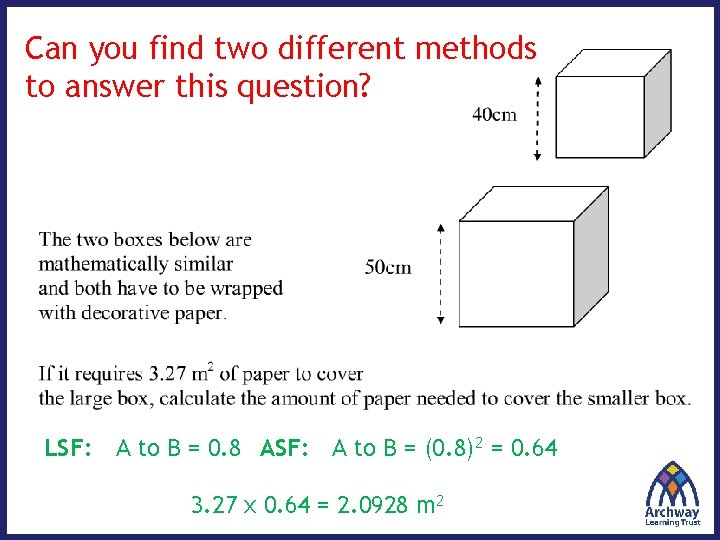Can you find two different methods to answer this question? LSF: A to B = 0. 8 ASF: A to B = (0. 8)2 = 0. 64 3. 27 x 0. 64 = 2. 0928 m 2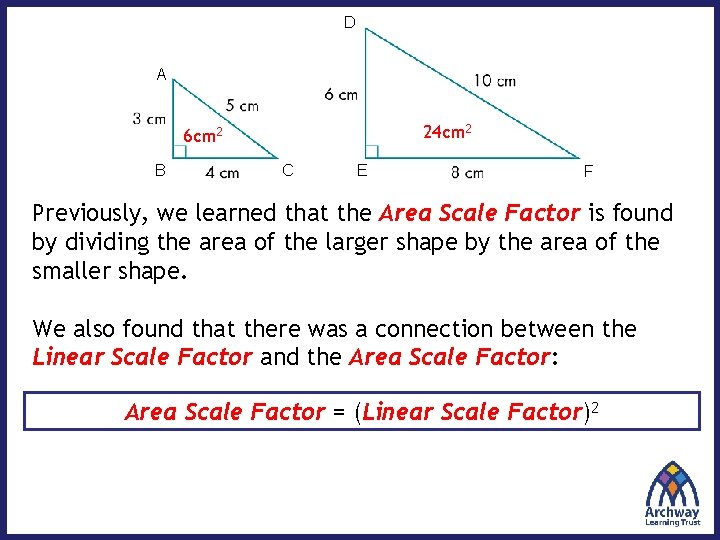D A 24 cm 2 6 cm 2 B C E F Previously, we learned that the Area Scale Factor is found by dividing the area of the larger shape by the area of the smaller shape. We also found that there was a connection between the Linear Scale Factor and the Area Scale Factor: Area Scale Factor = (Linear Scale Factor)2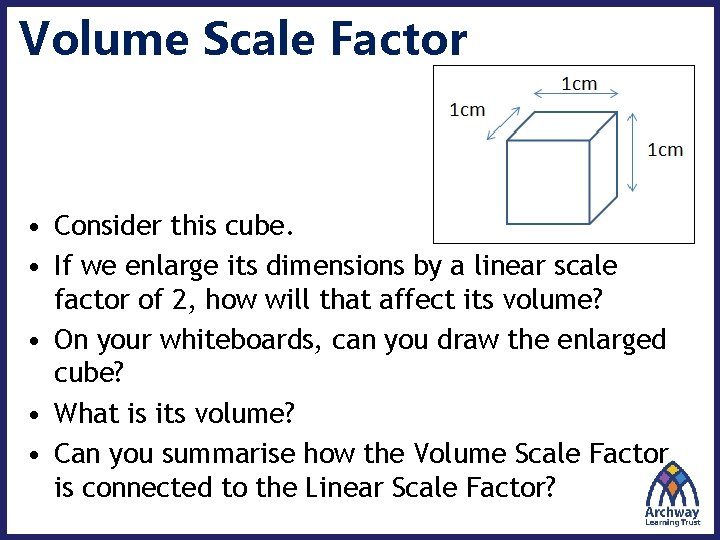Volume Scale Factor • Consider this cube. • If we enlarge its dimensions by a linear scale factor of 2, how will that affect its volume? • On your whiteboards, can you draw the enlarged cube? • What is its volume? • Can you summarise how the Volume Scale Factor is connected to the Linear Scale Factor?Volume Scale Factor How much bigger is Cube B compared to Cube A? m c 2 2 cm B 4 cm 2 cm A m c 4 4 cm A B Edge Length: 2 cm 4 cm Surface Area: 24 cm 2 96 cm 2 Total Volume: 8 cm 3 64 cm 3 SF 2 4 = 22 ? Knowing what you do about Length to Area Scale Factors, what can you say about extending this idea for the Volume Scale Factor?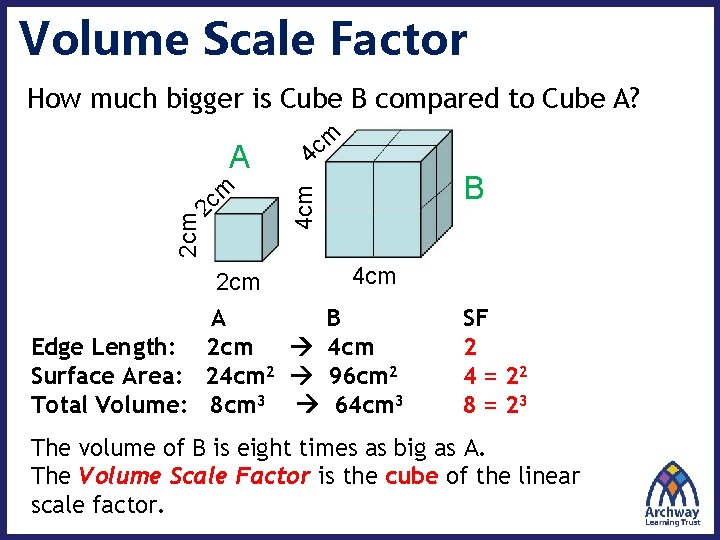Volume Scale Factor How much bigger is Cube B compared to Cube A? m c 2 2 cm B 4 cm 2 cm A m c 4 4 cm A B Edge Length: 2 cm 4 cm Surface Area: 24 cm 2 96 cm 2 Total Volume: 8 cm 3 64 cm 3 SF 2 4 = 22 8 = 23 The volume of B is eight times as big as A. The Volume Scale Factor is the cube of the linear scale factor.Volume Scale Factor (VSF): The proportional increase in the volume of two similar shapes Volume Scale Factor = (Linear Scale Factor)3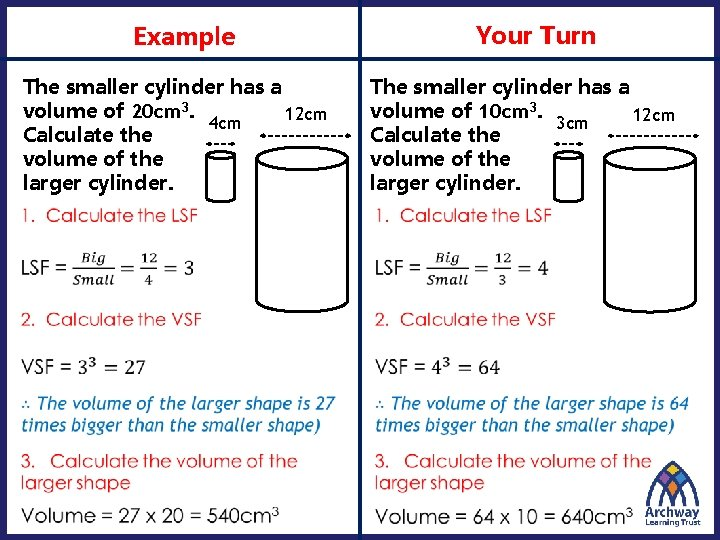Your Turn Example The smaller cylinder has a volume of 20 cm 3. 12 cm 4 cm Calculate the volume of the larger cylinder. The smaller cylinder has a volume of 10 cm 3. 12 cm 3 cm Calculate the volume of the larger cylinder.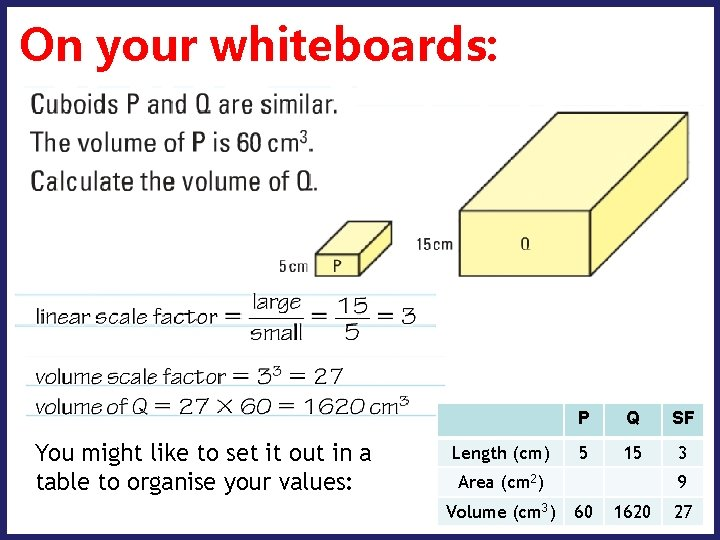On your whiteboards: You might like to set it out in a table to organise your values: Length (cm) P Q SF 5 15 3 Area (cm 2) Volume (cm 3) 9 60 1620 27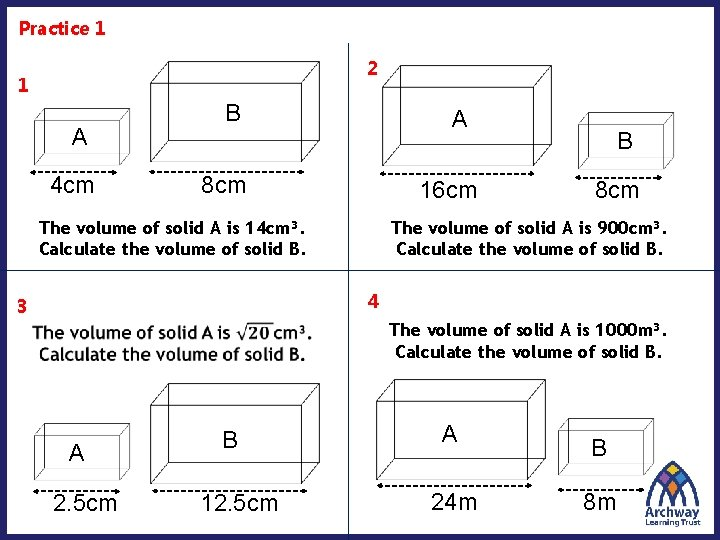Practice 1 2 1 A 4 cm B A 8 cm 16 cm The volume of solid A is 14 cm³. Calculate the volume of solid B. B 8 cm The volume of solid A is 900 cm³. Calculate the volume of solid B. 4 3 The volume of solid A is 1000 m³. Calculate the volume of solid B. A 2. 5 cm B 12. 5 cm A 24 m B 8 m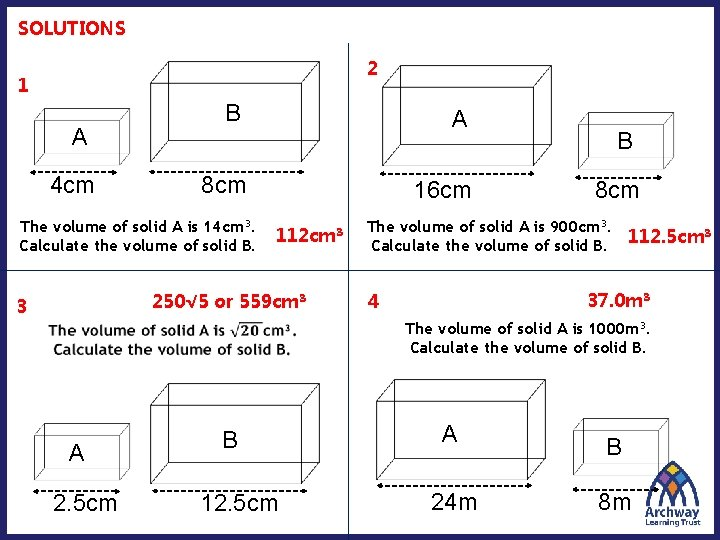SOLUTIONS 2 1 A 4 cm B A 8 cm The volume of solid A is 14 cm³. Calculate the volume of solid B. 16 cm 112 cm³ 250√ 5 or 559 cm³ 3 B 8 cm The volume of solid A is 900 cm³. Calculate the volume of solid B. 112. 5 cm³ 37. 0 m³ 4 The volume of solid A is 1000 m³. Calculate the volume of solid B. A 2. 5 cm B 12. 5 cm A 24 m B 8 m11 cm Finding the LSF: The smaller carton of double cream holds 125 ml, while the larger carton holds 200 ml. Assuming the dimensions of the two are similar, calculate the height of the larger carton.On your whiteboards: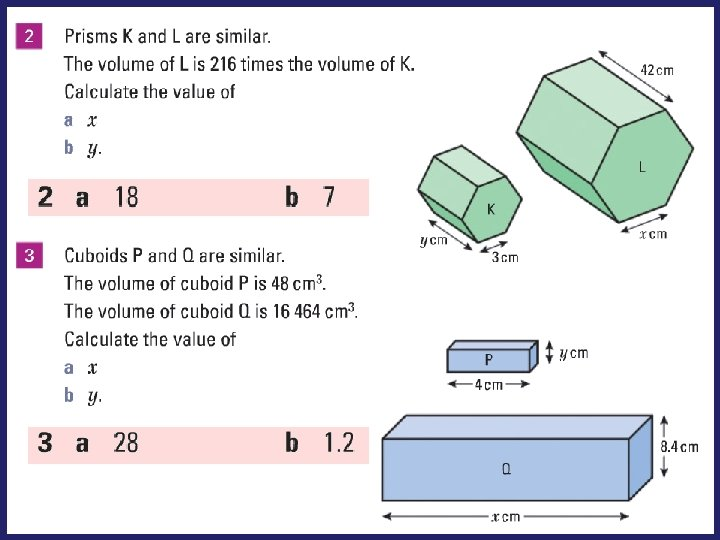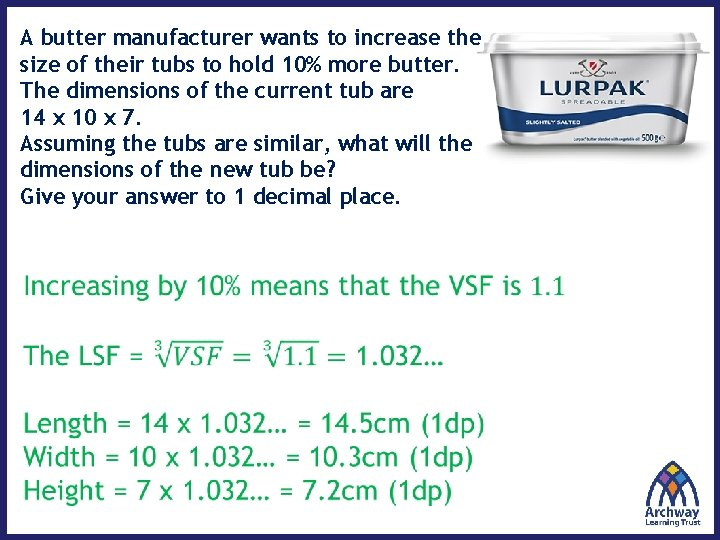A butter manufacturer wants to increase the size of their tubs to hold 10% more butter. The dimensions of the current tub are 14 x 10 x 7. Assuming the tubs are similar, what will the dimensions of the new tub be? Give your answer to 1 decimal place.A 1. 5 L bottle of Coca Cola has a height of 32 cm. If all bottles are geometrically similar, what are their heights? Give these measurements to 1 dp. Predict your answers before 33. 7 cm calculating them. 28. 0 cm 32 cm 22. 2 cm 35. 2 cm How accurate were your predictions? 500 ml 1 L 1. 5 L 1. 75 L 2 La Find the height of the larger cylinder b Find the area of the top of the larger cylinder. Give your answer in terms of π. c Find the volume of both cylinders. Give your answers in terms of π. Small Large SF Diameter (cm) 8 10 1. 25 Height (cm) 7 8. 75 1. 25 Area of top (cm 2) 16π 25π 1. 252 Volume (cm 3) 112π 218. 75π 1. 253